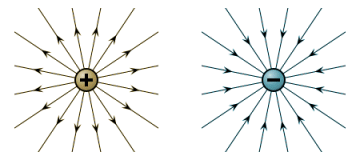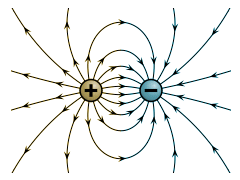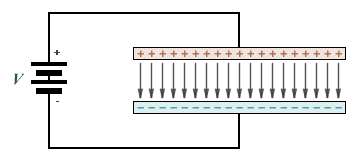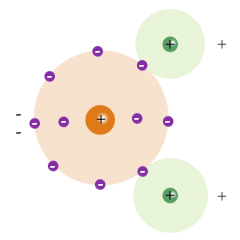# Electrostatics

## Overview

Electrostatics is a branch of science that is concerned with the effects of stationary (static) or slow-moving electrical charge that may exist in different types of materials. Under certain circumstances, charged particles can be transferred from one material to another leaving each with an overall positive or negative charge. Two materials that have the same net charge (i.e. they both carry a net overall positive charge or a net overall negative charge) will repel each other. Two materials that have a different net charge (i.e. one has a net overall positive charge while the other has a net overall negative charge) will attract one another. This attraction (or repulsion) is due to the electrostatic forces exerted by the charged particles on each other. The build up of charge on the surface of a particular material is known as static electricity.

Static electricity occurs when two different materials are brought into contact for a short time and then separated quickly. Because the two materials have different electrical properties, free electrons can migrate across the boundary between the two materials in one direction, creating a net positive charge on the surface of one material and a net negative charge on the surface of the other. If the materials are separated quickly enough, the electrons that have strayed across the boundary do not have sufficient time to get back. A net overall charge is left on both surfaces - one positive, and one negative (this is sometimes referred to as the triboelectric effect). The degree to which electrons will migrate across the boundary between two materials, and the speed with which they can get back again, depends on the combination of materials involved and will determine how much charge is transferred. Note that the overall charge for the system has not changed. There has simply been a transfer of charge from one material to the other (commonly referred to as the separation of charge).

The creation of a static charge occurs far more readily if at least one of the materials involved is a non-conducting material, because the charge built up on the surface of a non-conducting material cannot easily flow through the material and discharge itself to earth, and tends to be trapped there until it eventually bleeds away or is able to discharge itself by coming into contact with another (oppositely charged) material. Although simply putting two non-conducting materials together for a time and then separating them quickly can generate a static charge, rubbing the two materials together vigorously generates much larger amounts of static charge, because it increases the degree of surface contact and the number of separations that occur, providing greater opportunity for the exchange of charged particles. A classic example is rubbing a glass rod with silk, which causes electrons to move from the glass rod into the silk, and leaves the rod positively charged.

The Triboelectric Series is a list of materials ordered according to the electrical properties of the materials, and gives an indication of the type of charge (positive or negative) that will build up on the surface of the material when rubbed with (or placed in contact with) another material on the list. The materials near the top of the series will tend to acquire a positive charge, while those near the bottom will tend to acquire a negative charge. Note that when two different materials in the series are rubbed together, the one that is highest in the series will acquire the positive charge, while the lower ranked material will acquire the negative charge. The further apart the two materials are within the series, the greater the amount of charge generated. Two glass rods rubbed with silk will both acquire a positive charge and will therefore repel each other. Similarly, two Teflon rods rubber with rabbit fur will both acquire a negative charge and will repel each other. If one of the glass rods is brought into close proximity with one of the Teflon rods, however, they will be attracted to each other.

For each electron that migrates across the boundary between two materials, there will be an exchange of charge of the magnitude 1.6 × 10-19 coulombs (the coulomb is the SI unit of electrical charge). This charge is sometimes known as the elementary charge. A process known as charge induction can occur when a negatively charged object repels electrons from the surface of a second object creating a positively charged region in the second object. As a result, there will be an attraction between the two objects. The example you may have come across is that of rubbing a balloon on a woollen jumper. Since wool is much higher in the Triboelectric Series than rubber, the balloon acquires a negative charge. If you subsequently place the balloon against a wall, it will stay there for some time. The wall acquires a net positive charge at the surface immediately adjacent to the balloon due to charge induction, as free electrons at the surface are repelled by the balloon's negative charge.

Static electricity can be a hazard in some situations and of great benefit in others. Small electrical components can be damaged by electrostatic discharge, for example. Other hazards include the risk of electric shocks, and the generation of sparks which could ignite flammable gasses or liquids or even trigger an explosion. The many industrial and commercial applications of static electricity include industrial painting and coating systems, and photocopying equipment which uses electrostatic effects to deposit ink either directly onto paper or onto a rotating drum over which the paper will pass.

## Charge and electrostatic fields

An atom consists of a small central nucleus containing positively charged particles called protons, surrounded by an equal number of negatively charged (but much smaller) particles called electrons. The charge on a proton is equal in magnitude to that of an electron, so the atom does not normally have a net overall positive or negative charge. If an atom loses one or more electrons it becomes positively charged, because it will have more protons than electrons. If it gains one or more electrons it becomes negatively charged. An atom that carries a net positive or negative charge is called an ion. As mentioned above, the unit of charge is the coulomb which is defined as the quantity of electricity that flows past a given point in a circuit when a current of one ampere is maintained for one second (6.24 × 1018 electrons). We can express charge as follows:

Charge (Q) in coulombs  =  I  ×  t

Where:

I  =  the current in amperes

t  =  the time in seconds

Coulomb's law (named after French physicist Charles Augustin de Coulomb, who published it in 1783) describes the electrostatic force that exists between two charged particles (referred to as point charges ). Coulomb's law states:

"The force that one particle exerts on another particle is directly proportional to the product of their charges and inversely proportional to the square of their separation."

The force acting at any point for a single unit of charge is called the field strength E at that point, and is defined as the force (in newtons ) per unit charge (in coulombs ) that it would exert on a small positive test charge at that point. By convention, lines of force in an electric field are considered to act from positive to negative. The lines of force radiate outwards away from a positive charge, and inwards towards a negative charge as shown in the illustration below. The direction of the field at any point is taken to be the direction of the force acting on the positive test charge, and is therefore a vector quantity. When representing field strength in diagrams, the lines of force may be drawn closer together to indicate stronger electrostatic fields.Lines of force for positively and negatively charged particles

From Coulomb's law we know that a field generated by a point charge will exert a force on another charged particle. We know that the force exerted by the field can be expressed in newtons per coulomb (N C-1), and it can be shown that this is equivalent to a potential that can be expressed in volts per metre (V m-1). The magnitude of the electric field E created by a single point charge Q acting at a point d metres from the point charge (in free space) is:

 E  = Q 4πε0d2

Where ε0 is a constant called the permittivity of free space, and has a value of 8.854187817620 × 10-12 Nm3 / C. The illustration below shows the lines of force acting in the electrostatic field generated between two charged particles, one positive and one negative. For the force F exerted (in free space) by one charged particle Q1 on another charged particle Q2 when the distance between them is d metres, we get:

 F  = Q1Q2 4πε0d2The field surrounding two oppositely charged particles

We have already seen that the magnitude of the electric field E created by a single point charge Q acting at a point d metres from the point charge (in free space) is:

 E  = Q 4πε0d2

If the field is not acting in a vacuum, i.e. the intervening medium is air or some other material, the formula will change to the following:

 E  = Q 4πε0εrd2

Where:

εr  =  the relative permittivity of the medium

This will similarly affect our calculation of the force F exerted by one charged particle Q1 on another charged particle Q2 when the distance between them is d metres. If the material separating the two charges is anything other than a vacuum, we get:

 F  = Q1Q2 4πε0εrd2

## The electrostatic field between two charged plates

An electric field will exist between two oppositely charged parallel plates such that lines of force will act in the perpendicular direction from the positively charged plate to the negatively charged plate, as illustrated below. We will assume for the purposes of this discussion that the plates are separated by air or some other non-conducting material, and that the charge is due to an applied voltage V. A negatively charged particle located between the plates will experience a force that attempts to push it in the direction of the positively charged plate (a positively charged particle would experience an equal force in the opposite direction). However, because the material between the plates is non-conducting there will be no significant migration of charge.Lines of force between two oppositely charged parallel plates

The imaginary lines of force drawn on diagrams such as the one above are used to represent the flux of the system. Charge and flux are closely related, since the charge creates the electric field that is responsible for the flux. Both charge and flux are scalar quantities (i.e. they have magnitude but not direction). Flux is the amount of something passing through a surface at a given point in time. In this case, the "something" is the electric field generated by a charge. This concept was formalised by Carl Friedrich Gauss in 1867 as Gauss's Law, which states:

"The electric flux through any closed surface is proportional to the enclosed electric charge."

The electric flux Φ through a given surface can be defined as the electric field E multiplied by the area A of the surface projected in a plane perpendicular (at right angles) to the field. This can be formalised as:

Φ  =  EA cos (θ)

Where θ is the angle between the field and the normal of the surface.

The diagram below illustrates the principle, and shows the electric field acting through a surface at angle θ to the normal. Note that if the field is perpendicular to the surface in question, angle θ will be zero and the value of cos (θ) will be 1, simplifying the calculation.An electric field acting through a surface

If the flux acting through a surface can be thought of as the total number of lines of force acting through a given surface area, then the flux density can be thought of as the number of lines of force per unit area. Taking our charged parallel metal plates as an example, if the charge on each plate is uniformly distributed across its surface area A, then the flux density D can be calculated as:

 D  = Φ A

It can also be shown that the flux passing through a given surface is equal to the charge on the surface divided by the permittivity of free space, expressed as follows:

 Φ  = Q ε0

Since the charge density σ on the surface will be the total charge divided by the area of the surface we can express charge as:

Q  =

Therefore:

 Φ  = Aσ ε0

In the case of our charged parallel plates, the field will be acting perpendicular to the surface of the plate and the total flux will be given by:

Φ  =  EA

From the above we get:

 E  = Φ = 1 × Aσ = σ A A ε0 ε0

In other words, the magnitude of the electric field at the surface of the conductor will be equal to the charge density divided by the permittivity of free space. The electric field strength E between the two plates will depend on the applied voltage V and the distance d (in metres) between the plates, and is given by:

 E  = V d

## Dielectric materials

The insulating material between two conducting surfaces is called a dielectric. It may be a vacuum, air, or one of many non-conducting materials. With the exception of gases, all non-conducting materials increase the capacitance (the ability to store charge) of the surfaces. The degree to which the dielectric material increases capacitance can be determined from its dielectric constant. If the surfaces are separated by a material with a dielectric constant of 2, the capacitance will be two times greater than it would be if the surfaces were separated by air or a vacuum. Note that if the voltage is increased sufficiently, any dielectric material can be forced to conduct electricity and will suffer dielectric breakdown.

All dielectric materials will become polarised when in close proximity to a charged object. The material will not acquire a significant overall charge, since the electrons in a non-conducting material tend to be tightly bound to their atoms. However, the electrons within the atoms making up the dielectric material will re-arrange themselves under the influence of the electric field created by an external charge, as shown below. The degree to which polarisation occurs will determine the material's dielectric constant.Un-polarised atom (left) and polarised atom (right)

You can see from the diagram that the atom has been stretched by the polarisation. The positively charged nucleus is displaced in the direction of the field, while he negatively charged electrons are displaced in the opposite direction. The atom effectively becomes a dipole, with its own internal electric field that partially compensates for the external electric field. Some materials (water, for example) have a molecular structure which is already polarised. Oxygen has six valence electrons in its outer shell, which means it is two electrons short of a full shell. When two hydrogen atoms bond with one oxygen atom to form a water molecule, each hydrogen atom effectively donates its single electron to complete the outer shell of the oxygen atom, as shown below. The result is a net positive charge on the on the hydrogen atoms, and a net negative charge on the oxygen atom. The application of an external field to polar molecules will increase the degree of polarisation through stretching, and in weakly bonded molecules may cause a re-alignment (or rotation) of the molecule itself whereby its polar axis aligns itself in the direction of the field.A polar water molecule is effectively a dipole